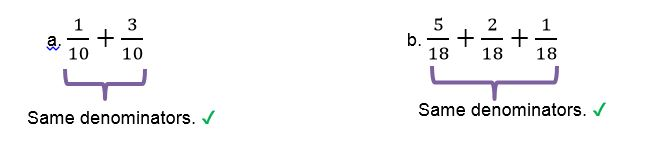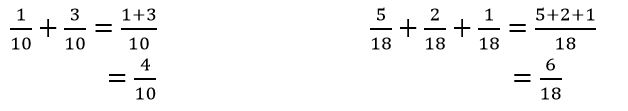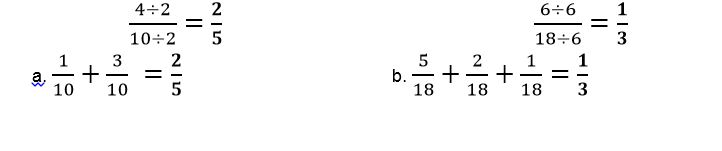# How to add fractions with common denominators

Want to find out how to add fractions with common denominators? We've provided a step-by-step guide of how to add fractions with common denominators to teach you everything you need to know.## Step 1: Confirm denominators are common

Check the fractions’ denominators are the same.

## Step 2: Add the numerators directly

As the fractions have the same denominators, you can add them directly. The denominator remains the same with that of the given fractions.

## Step 3: Simplify the fraction with a common factor

If you can, divide both the numerator and denominator by the common factor.

## Examples on adding fractions with common denominators

Q1) Find the sum of the following sets of fractions:Add the numerators and write down the common denominator.Simplify the sum by finding the common factors.#### Join today

The fastest way to practice

Unlock our complete testing platform and improve faster that ever.